# 2. Data setThe data set contains information for creating our model. It is a data collection structured as a table in rows and columns.

The most popular data set in the machine learning field is the Iris flower data set. The British statistician and biologist Ronald Fisher introduced this data set in 1936.

We can identify the next concepts in a dataset:

## 2.1. Data source

The data is usually stored in a data file. Some common data sources are the following:Datafiles
• CSV.
• Excel.
• OpenOffice Calc.
• Etc.

From all that, the most used format for a data set is a CSV file. When possible, export to CSV your spreadsheet file, SQL query, etc.

## 2.2. Variables

The variables are the columns in the data table. Variables might represent physical measurements (temperature, velocity,…), personal characteristics (gender, age,…), marketing dimensions (recency, frequency, monetary,…), etc.

Regarding their use, we can talk about:

### Input variables

Input variables are the independent variables in the model. They are also called features or attributes.

Input variables can be continuous, binary, or categorical.

### Target variables

Target variables are the dependent variables in the model.

In regression problems, targets are continuous variables (power consumption, product quality…).

In classification problems, targets are binary (fault, churn…) or categorical (the type of object, activity…). In this type of application, targets are also called categories or labels.

### Unused variables

Unused variables are neither inputs nor targets. We can set a variable to Unused when it does not provide any information to the model (id number, address, etc.).

Constant variables are those columns in the data matrix, always having the same value. They should be set as Unused since they do not provide any information to the model but increase its complexity.

## 2.3. Samples

Samples are the rows in the data table. They are also called instances or points.

It is not helpful to design a neural network to memorize a set of data simply. Instead, we want the neural network to perform accurately on new data, that is, to generalize.

To achieve that, we divide the data set into different subsets:

### Training samples

During the design of a model, we usually need to try different settings. For instance, we can build several models with different architectures and compare their performance. To construct all these models, we use the training samples.

### Selection samples

Selection samples are used for choosing the neural network with the best generalization properties. In this way, we construct different models with the training subset and select the one that works best on the selected subset.

### Testing samples

Testing samples are used to validate the functioning of the model. We train different models with the training sampless, select the one that performs best on the selected samples, and test its capabilities with testing samples.

### Unused samples

Some samples might distort the model instead of providing helpful information to the model. For example, outliers in the data can make the neural network work inefficiently. To fix these problems, we can set those samples to Unused.

The standard is to use 60% of the samples for training, 20% for selection, and 20% for testing. Splitting of the samples might be performed in sequential order or randomly.

We can also set repeated samples to Unused since they provide redundant information to the model.

## 2.4. Missing values

A data set can also contain missing values, which are those elements that are not present.
Usually, missing values are denoted by a label in the data set. Some standard labels used for representing missing values are NA (not available), NaN (not a number), Unknown, or ?. Do not use numeric values here, such as -999, since that might be confused with an actual value.

There are two ways to deal with missing values:

### Missing values unusing

If the number of samples in the data set is significant and the number of missing values is small, we can exclude those samples with missing values from the analysis.

In this way, the unusing method sets those samples with missing values to Unuse.

### Missing values imputation

If the data set is small or the number of missing values is significant, you probably cannot afford to unuse the samples with missing values. In these cases, assigning probable values to the missing data is advisable.

The most common imputation method is to substitute the missing values with the mean value of the corresponding variable.

Some of the essential techniques for data analysis and preparation are the following:

### Statistics

Basic statistics are precious information when designing a model since they might alert on the presence of spurious data. It is necessary to check for the correctness of every variable’s most critical statistical measures.

As an example, the following table depicts the minimum, maximum, mean, and standard deviation of the variables used to improve the performance of a combined cycle power plant.

MinimumMaximumMeanStandard deviation
temperature1.8137.1119.657.45
exhaustt_vacuum25.3681.5654.3112.71
ambient_pressure992.891033.301013.265.94
relative_humidity25.56100.1673.3114.60
energy_output420.26495.76454.3717.07

In classification applications, it is also interesting to compare the statistics for each category. For instance, we can compare the mean age of customers who buy a particular product with the mean age of customers who do not.

### Distributions

Distributions show how the data is distributed over its entire range. If the data is very irregularly distributed, the resulting model will probably be of poor quality.

Histograms are used to see how continuous variables are distributed. Continuous variables usually have a normal (or gaussian) distribution.

For example, the following figure depicts a histogram for the noise generated by different airfoil blades.As we can see, this variable has a normal distribution. 22% of the airfoil blades in the data set emit a sound around 127 dB.

Pie charts are used to see the distribution of binary or nominal variables. This type of variable should be uniformly distributed.

The following figure shows the pie chart for the customers of a bank that purchase a bank deposit in a marketing campaign, which is a binary variable.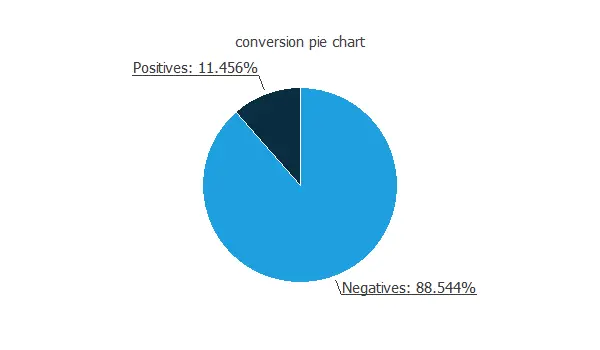Approximately 85.5% of the customers do not purchase the product, 14.5% purchase it. Therefore, this variable is not well-balanced, which means many more negatives than positives.

### Box plots

Box plots also provide information about the shape of the data. They display information about every variable’s minimum, maximum, first quartile, second quartile (or median), and third quartile. They consist of two parts: a box and two whiskers.

The length of the box represents the interquartile range (IQR), which is the distance between the third quartile and the first quartile. The middle half of the data falls inside the interquartile range. The whisker below the box shows the minimum of the variable. On the other hand, the whisker above the box shows the variable’s maximum.
Within the box, we also draw a line that represents the median of the variable.

The following figure illustrates the box plot for the age of the employees of a company.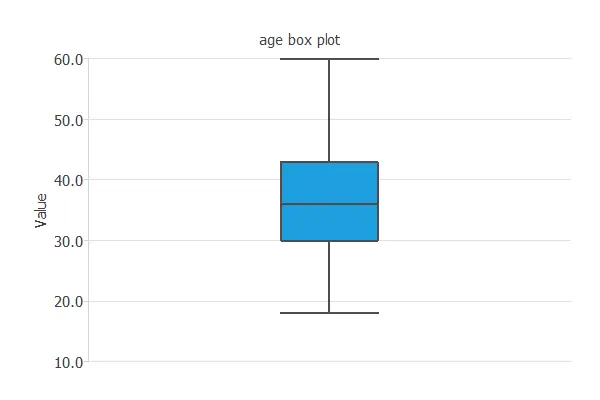As we can see, 50% of the customer are between 35 and 45 years old.

### Time series plots

It is helpful to see how the different variables evolve over time in forecasting applications. In this way, time series plots display observations in the y-axis against time in the x-axis.

The following figure is an example of a time series plot. It depicts the temperature in a city over the years.The figure from above shows a cyclic variable. It also shows some outliers in the data (wrong temperature observations).

### Scatter charts

It is always beneficial to see how the targets depend on the inputs. Scatter charts show points with target values versus input values.

For example, the following chart shows concretes compressive strength as a function of cement quantity.
As we can see, there is quite a strong correlation between both variables: the more quantity of cement, the more compressive strength.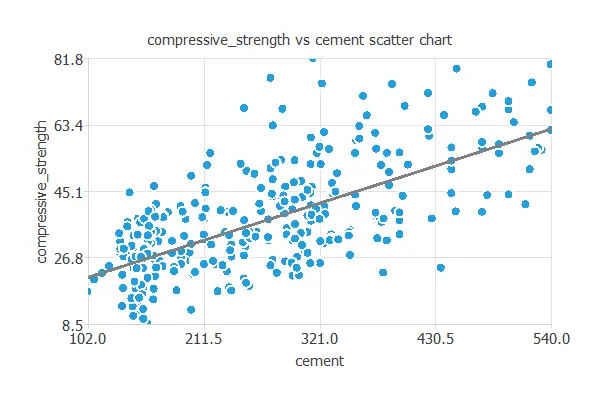### Inputs correlations

Sometimes, data sets contain redundant data that complicate the design of the neural network. To discover redundancies between the input variables, we use a correlation matrix.

The correlation is a numerical value between -1 and 1 that expresses the strength of the relationship between two variables. When it is close to 1, it indicates a positive relationship (one variable increases when the other increases); a value close to 0 indicates no relationship; a value close to -1 indicates a negative relationship (one variable decreases when the other decreases).

We calculate the correlation using a linear function when both variables are continuous. When one or both variables are binary, we calculate the correlation using a logistic function.

Next, we depict the correlations among the features used to target donors in a blood donation campaign. This example uses a recency, frequency, monetary, and time (RFMT) marketing model.

RecencyFrequencyQuantityTime
Recency1.00-0.18-0.180.16
Frequency 1.001.000.63
Quantity  1.000.63
Time   1.0

In this case, the quantity variable has a perfect correlation with the frequency variable (1.00). That means that we can remove one of those two variables in our model without losing information.

### Inputs-targets correlations

It is beneficial to know the dependencies of the target variables with the input variables. For this kind of diagnostic analytics, we also use correlation coefficients. If the correlation is 0, they are independent of each other. Increasing or decreasing one does not imply that it increases or decreases another. On the other hand, if the correlation coefficient is 1 or -1, they are directly or inversely dependent.

We use linear correlations when both the input and the target are continuous. We use logistic correlations when one or both the input and the target are binary.

The following figure shows the correlations between the dimensions and velocity of a sailing yacht and its corresponding hydrodynamic performance.

From the above figure, we can see that a variable with a high correlation (more than 0.5) and about ten variables with a small correlation (less than 0.1).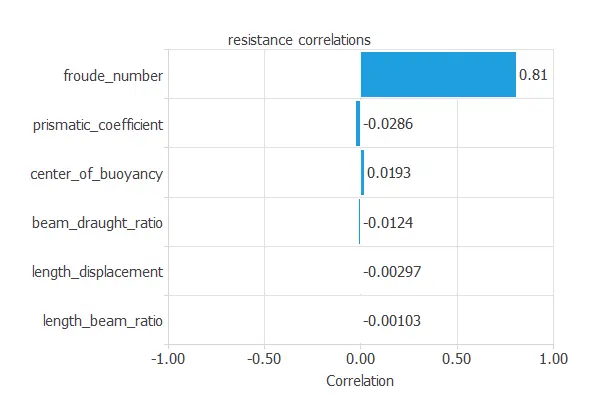### Autocorrelations

In forecasting applications, autocorrelations refer to the correlations of a time series with its past values.

We call positive autocorrelated time series persistent because positive deviations from the mean follow positive deviations from the mean. Conversely, negative deviations from the mean follow negative deviations from the mean.

On the other hand, negative autocorrelated time series are characterized by a tendency for positive deviations from the mean to be followed by negative deviations from the mean and vice-versa.

The following figure shows an example of an autocorrelations chart. It depicts the correlations among meteorological variables from the past five days.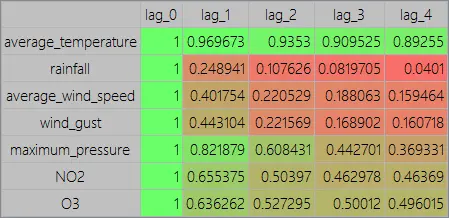As we can see, the highest autocorrelated variable is the temperature, and the least autocorrelated variable is the rainfall.

### Cross-correlations

In forecasting applications, cross-correlation charts show the correlation between a target variable and the lags of an input variable.

As an illustration, the following figure shows the correlations between the rainfall of today and the maximum pressure of yesterday, the day before yesterday, etc.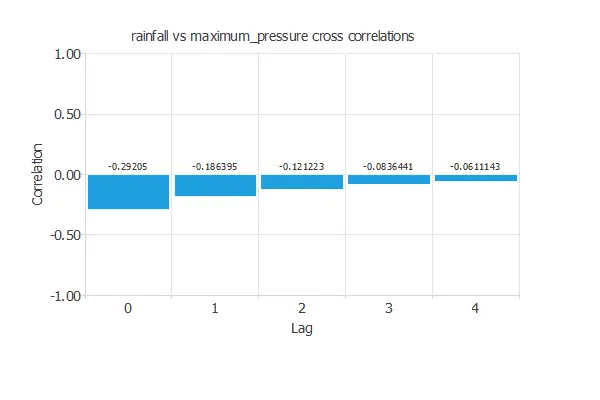The chart above shows negative and decreasing correlations.

### Outliers

An outlier is an sample that is distant from other samples. They may be due to variability in the measurement or may indicate experimental errors.

If possible, we should exclude outliers from the data set, setting that samples as Unused. However, detecting those anomalous samples might be difficult and usually requires lots of work.

The first thing we can do is to check for the correctness of the data statistics. Indeed, spurious minimums and maximums are a clear sign of the presence of outliers.

We can also plot the data histograms and check that there are no isolated bins at the ends.

Box plots are also suitable for detecting outliers’ presence since they depict data groups through their quartiles.

In this regard, Tukey’s method defines an outlier as those values of the data set that fall too far from the central point, the median. The cleaning parameter defines the maximum distance to the center of the data that will be allowed. As it grows, the test becomes less sensitive to outliers, but many values will be detected as outliers if it is too small.

For example, the following chart is a box plot of the balance of a bank’s customers. As we can see, there are a few clients with a very high balance, and we can treat them as outliers.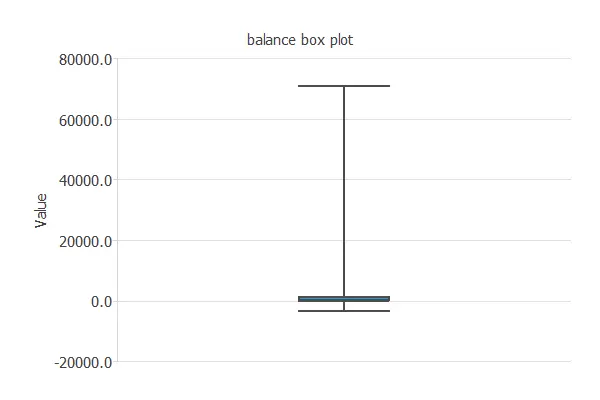There are other methods for dealing with outliers, such as the Minkowski error. For more information, you can read the 3 methods to deal with outliers post in our blog.

### Filtering

The objective of filtering is to reduce the noise or errors in the data.

Here the samples that do not fall in a specified range are unused.

When filtering data, the minimum and maximum allowed values for all the variables must be set.

### Uncorrelated variables unusing

Unusing uncorrelated variables allows reducing the problem dimensions without much loss of information.